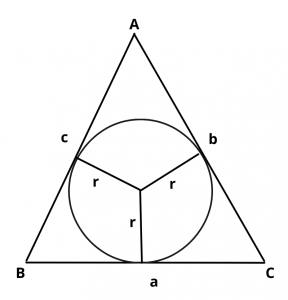# What is the Formula for the Inradius ?

## Solution :

Inradius Formula (r) = $$\Delta\over s$$Where r = radius of the circle inscribed in a given triangle

$$\Delta$$ = area of the given triangle

$$\Delta$$ = $$\sqrt{s(s – a)(s – b)(s – c)}$$

s = half perimeter of the given triangle

s = $$a + b + c\over 2$$  for all a, b c are the sides of a given triangle.